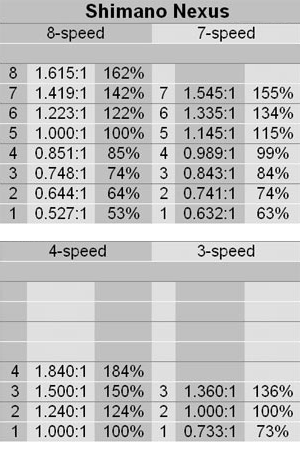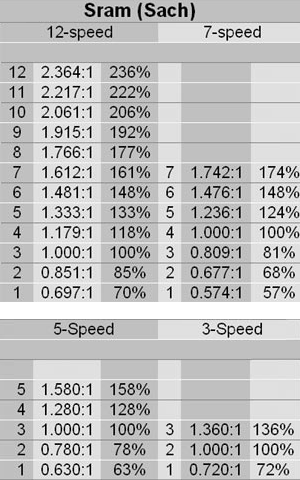# How fast? at what gear ratio? here's how-

2 posts / 0 new
chas_stevensonOffline
Last seen: 8 years 11 months ago
Joined: Wednesday, December 6, 2006 - 17:14
Points: 1309
How fast? at what gear ratio? here's how-

--- Calculating Gear Ratios ---

If you know how fast you want to go, the diameter of the drive tire/wheel, and the loaded RPM of your motor you can calulate the gear ratio needed to go that fast.Gear ratios can be calculated by taking the wheel rollout (how far the wheel moves in 1 rotation) dividing this amount into the distance to be used, Miles or Kilometers. Then to get the required gear ratio, take the Motor RPM and divide it by the RPM at a given speed.

This example will use inches and miles to get MPH and calculate the required gear ratio.
Note: I am using the wheel size as the wheel diameter. To be more accurate, measure the diameter of the wheel you will be using.

Rollout for a wheel: WD x pie = D
Where: WD = Wheel Diameter and pie = 3.1428
D is the distance the wheel travels in 1 rotation.

26 inch wheel = 81.71 inches
20 inch wheel = 62.86 inches
16 inch wheel = 50.29 inches

Now we need to find out how many times the wheel must rotate to travel 1 mile.
There are 63360 inches in 1 mile: 63360 / D = RPH
Where D is the distance the wheel travels in 1 rotation.
RPH = Rounds Per Hour

26 inch wheel = 775.43 Rotations to travel 1 mile.
20 inch wheel = 1007.95 Rotations to travel 1 mile.
16 inch wheel = 1259.89 Rotations to travel 1 mile.

To get the RPM we must divide the rotations by 60 for 60 minutes in 1 hour.
RPH / 60 = RPM
RPM = Rounds Per Minute

26 inch wheel = 12.92 Rotations to travel 1 mile per hour.
20 inch wheel = 16.80 Rotations to travel 1 mile per hour.
16 inch wheel = 21.00 Rotations to travel 1 mile per hour.

Multiply this by the desired speed and you have the max RPM the wheel needs to turn to achieve the required speed.

This example will use 20MPH.: RPM x MPH = RPM@speed

26 inch wheel = 258.4 Rotations Per Minute to travel 20 mile per hour.
20 inch wheel = 336.0 Rotations Per Minute to travel 20 mile per hour.
16 inch wheel = 420.0 Rotations Per Minute to travel 20 mile per hour.

If we know the RPM of the motor we can calculate the gear ratio.

Example:

For a 2400-RPM motor and a 26-inch wheel the ratio would be 2400 / 258.4 = 9.29 to 1
For a 2400-RPM motor and a 20-inch wheel the ratio would be 2400 / 336.0 = 7.14 to 1
For a 2400-RPM motor and a 16-inch wheel the ratio would be 2400 / 420.0 = 5.71 to 1

For a 3000-RPM motor and a 26-inch wheel the ratio would be 3000 / 258.4 = 11.6 to 1
For a 3000-RPM motor and a 20-inch wheel the ratio would be 3000 / 336.0 = 8.93 to 1
For a 3000-RPM motor and a 16-inch wheel the ratio would be 3000 / 420.0 = 7.14 to 1

This should give you a good idea of how you can calculate your gear ratios.

chas_stevensonOffline
Last seen: 8 years 11 months ago
Joined: Wednesday, December 6, 2006 - 17:14
Points: 1309
Internally Geared Hubs

For anyone wanting to know the gear ratios of the Internal Geared Hubs here are charts for the most common hubs.## Who's online

There is currently 1 user online.

• flapjacksbestof